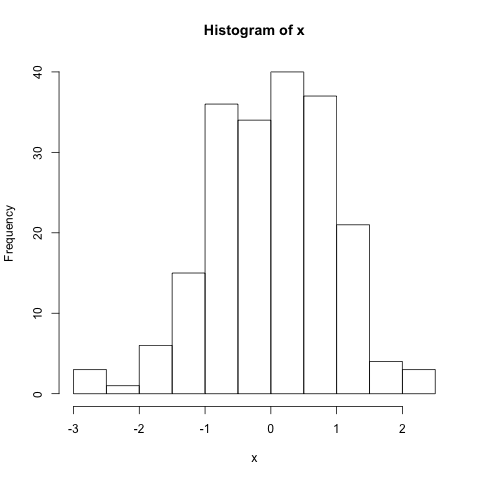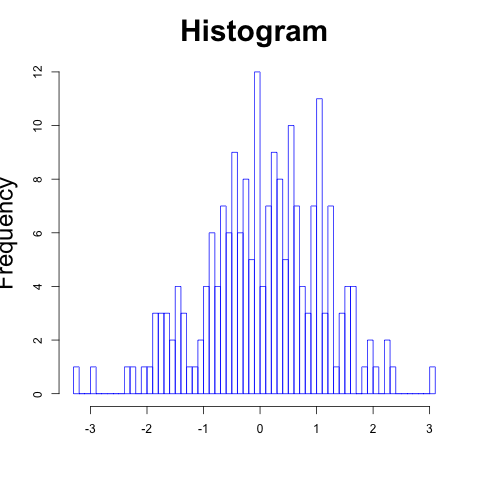## 16.5 Histograms

A histogram graphically summarizes the distribution of the data.

• A simple histogram
``````# Vector of 200 random values from the normal distribution
x <- rnorm(200)

# Plot histogram
hist(x)````````````hist(x,